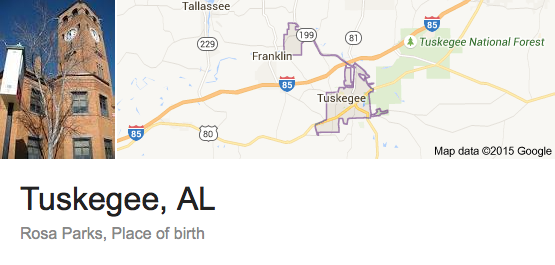## Wednesday, April 29, 2015

### 24 42 59 93 | Rosa Parks, On the Bus, by the Numbers... and Outkast's Lawsuit

Notice below, Rosa was '42' at the time of the 'bus' protests.

Bus = 2+21+19 = 42

• Rosa = 9+6+1+1 = 17/26
• Louise = 3+6+3+9+1+5 = 27/36
• McCauley = 4+3+3+1+3+3+5+7 = 29
• Parks = 7+1+9+2+1 = 20/29
• Rosa Louise McCauley Parks = 93/120
Do you notice anything curious about Rosa Parks birthday?  It is February 4.  You could argue this is the most "Nigger Day" on the calendar.  I don't mean that in an offensive way, only in an educational way.  For this lesson is about seeing through the Zionist assholes who rig our world by the numbers.
• Nigger = 5+9+7+7+5+9 = 42
• February = 6+5+2+9+3+1+9+7 = 42
• Think about the date February 4, or 2/4, or often written 4/2
• February is black history month...
• Black = 2+3+1+3+2 = 11
• History = 8+9+1+2+6+9+7 = 42
• Black History = 53
• February 4 is the 35th day of the year
Rosa barks is best remembered for what she did on the 'bus'.
• Bus = 2+21+19 = 42
Notice that in 1942. Rosa Parks followed in the footsteps of Bayard Rustin, who started his protest in that special year.

• Bayard = 2+1+7+1+9+4 = 24
• Rustin = 9+3+1+2+9+5 = 29
• Bayard Rustin = 53
• Bayard's action were also on a bus.
• So were Irene Morgan's
• Irene = 9+9+5+5+5 = 33 (Race War = 9+1+3+5+5+1+9 = 33)
• Morgan = 4+6+9+7+1+5 = 32
• Irene Morgan = 65Rosa Parks place of birth.
• Tuskegee = 2+3+1+2+5+7+5+5 = 30/39
• Tuskegee = 20+21+19+11+5+7+5+5 = 93
Rosa Parks died on October 24, 2005.
• 10/24/05 = 10+24+05 = 39 (Tuskegee = 39)(Her birth town)
• 10/24/2005 = 10+24+20+05 = 59
• Negro = 59
• Slave = 59
• Blues = 59
• Miles Davis = 59
• Miles Davis came out in '59
• Motown came out in '59
• Lynching of Mr. Parker (Parker = 33/42) in '59
Curious date, wouldn't you say?  Notice that she died on the 24th day, the reflection of 42, and in the only month with '33' Gematria, October.  She died in a '7' year, 2+0+0+5.

She is remembered for her actions in Montgomery, Alabama, in the bus, on December 1, 1955.
• 12/1/1955 = 1+2+1+1+9+5+5 = 24
• 12/1/55 = 12+1+55 = 68 (Montgomery, Alabama = 68)
• Montgomery = 4+6+5+2+7+6+4+5+9+7 = 55
• Alabama = 1+3+1+2+1+4+1 = 13
• Montgomery, Alabama = 68
Outkast Lawsuit-
The Rap Group Outkast did a song about Rosa Parks and was sued by her.  Both members of that rap due are named 'Andre'.

#### 1 comment:

1.She was 42 years old at the time of the bus thing as well.